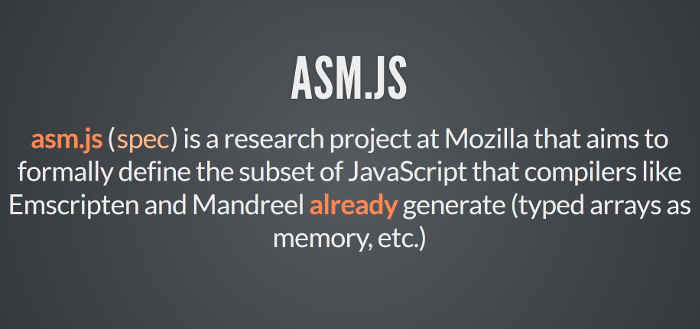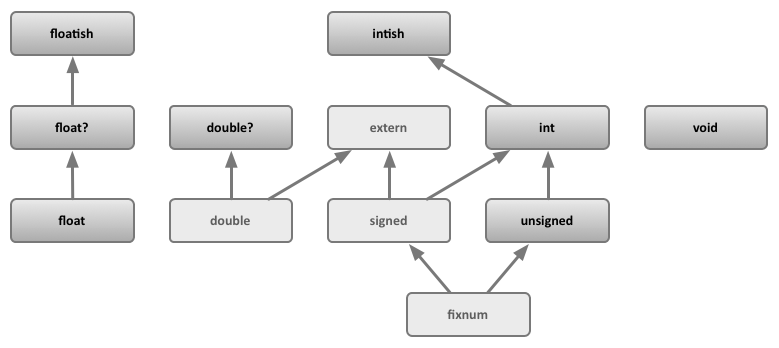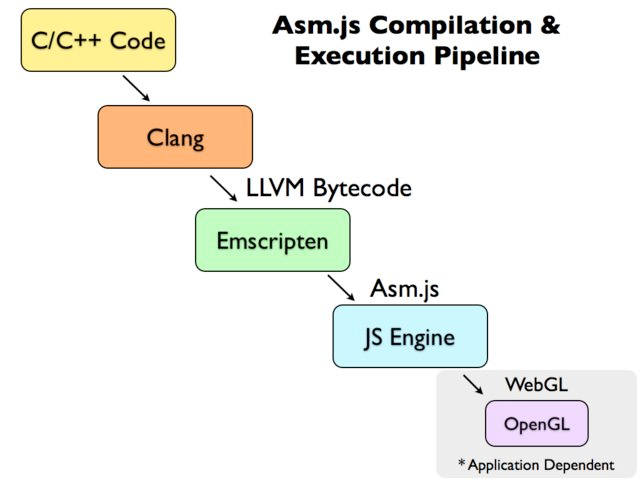Web technology has advanced by leaps and bounds, but there is one area that has been impossible to break through —- games.

The performance requirements for games are so high that some large games struggle to run even on a PC, let alone in the sandbox model of a browser! However, despite the difficulties, many developers have never given up and want to make browsers run 3D games.In 2012, Alon Zakai, an engineer at Mozilla, was working on the LLVM compiler and had the idea that many 3D games are written in C/C++, so if we could compile C/C++ into JavaScript code, they would run in the browser. As we all know, the basic syntax of JavaScript is highly similar to C language.

So, he started working on how to achieve this goal and made a compiler project called Emscripten, which compiles C/C++ code into JS code, but not just any JS, but a variant of JavaScript called asm.js.

In this article, we will introduce the basic usage of asm.js and Emscripten, and describe how to convert C / C++ to JS.## Introduction to asm.js

### Principle

There are two biggest difficulties with compiling C / C++ to JS.

• C / C++ is a statically typed language, while JS is a dynamically typed language.
• C / C++ is manual memory management, while JS relies on garbage collection mechanisms.

asm.js is designed to solve these two problems: its variables are always statically typed and it does away with the garbage collection mechanism. Other than these two points, it is not different from JavaScript, i.e. asm.js is a strict subset of JavaScript and can only use part of the latter’s syntax.Once the JavaScript engine finds out that it is running asm.js, it knows that it is optimized code and can skip the syntax analysis step and go straight to assembly language. In addition, the browser calls WebGL to execute asm.js through the GPU, meaning that asm.js has a different execution engine than a normal JavaScript script. These are the reasons why asm.js runs faster. It is claimed that asm.js runs in the browser at about 50% of the speed of native code.

The two main syntactic features of asm.js are described below in turn.

### Static type variables

asm.js provides only two data types.

• 32-bit signed integer
• 64-bit Signed Floating PointOther data types, such as strings, booleans or objects, are not provided by asm.js at all. They are stored in memory as numeric values and called via TypedArray.

If the type of a variable is to be determined at runtime, asm.js requires that the type be declared beforehand and not changed, thus saving time in type determination.

The type declaration in asm.js is written in a fixed way, with `Variable | 0` for integers and `+Variable` for floating point numbers.

 ``````1 2 3 4 `````` ``````var a = 1; var x = a | 0; // x is a 32-bit integer var y = +a; // y is a 64-bit floating point number ``````

In the above code, the variable `x` is declared as an integer and `y` is declared as a floating point number. An engine that supports asm.js will know that `x` is an integer when it sees `x = a | 0`, and will use the asm.js mechanism to handle it. If the engine doesn’t support asm.js, it doesn’t matter, the code will still work and you’ll get the same result.

Look at the following example.

 ``````1 2 3 4 5 6 7 `````` ``````// Writing Method 1 var first = 5; var second = first; // Writing Method 2 var first = 5; var second = first | 0; ``````

In the above code, write 1 is normal JavaScript, the variable `second` only knows the type at runtime, which is slow, write 2 is asm.js, `second` is known to be an integer at declaration time, which is faster.

The arguments and return values of the function, both have to specify the type in this way.

 ``````1 2 3 4 5 6 `````` `````` function add(x, y) { x = x | 0; y = y | 0; return (x + y) | 0; } ``````

In the above code, in addition to the arguments `x` and `y`, the return value of the function also needs to have its type declared.

### Garbage collection mechanism

asm.js has no garbage collection mechanism and all memory operations are controlled by the programmer. asm.js reads and writes memory directly through TypedArray.

Here is an example of reading and writing memory directly.

 ``````1 2 3 4 5 6 `````` ``````var buffer = new ArrayBuffer(32768); var HEAP8 = new Int8Array(buffer); function compiledCode(ptr) { HEAP[ptr] = 12; return HEAP[ptr + 4]; } ``````

If a pointer is involved, it is handled the same way.

 ``````1 2 3 4 5 6 7 8 `````` `````` size_t strlen(char *ptr) { char *curr = ptr; while (*curr != 0) { curr++; } return (curr - ptr); } ``````

The above code is compiled into asm.js, which looks like the following.

 ``````1 2 3 4 5 6 7 8 9 `````` ``````function strlen(ptr) { ptr = ptr|0; var curr = 0; curr = ptr; while (MEM8[curr]|0 != 0) { curr = (curr + 1)|0; } return (curr - ptr)|0; } ``````

### Similarities and differences between asm.js and WebAssembly

If you’re familiar with JS, you may know that there is a technology called WebAssembly that also converts C/C++ into code that can be run by JS engines. So what’s the difference between it and asm.js?

The answer is that they basically do the same thing, but the code that comes out is different: asm.js is text and WebAssembly is binary bytecode, so it runs faster and is smaller. In the long run, the future of WebAssembly is brighter.

However, this does not mean that asm.js is definitely out, because it has two advantages: first, it is text, human readable and more intuitive; second, all browsers support asm.js and there are no compatibility issues.

## Emscripten Compiler

### Emscripten Introduc

Although asm.js can be written by hand, it is never the target language of the compiler and has to be generated by compilation. Currently, the main tool for generating asm.js is Emscripten.The underlying layer of Emscripten is the LLVM compiler, and in theory any language that can generate LLVM IR (Intermediate Representation) can be compiled to generate asm.js. In practice, however, Emscripten is almost exclusively used to compile C/C++ code to generate asm.js.

 ``````1 `````` ``````C/C++ ⇒ LLVM ==> LLVM IR ⇒ Emscripten ⇒ asm.js ``````### Installation of Emscripten

The installation of Emscripten can be done according to the official documentation. As there are many dependencies and it is a bit tricky to install, I found a more convenient way is to install the SDK.

You can follow the steps below.

 ``````1 2 3 4 5 `````` ``````\$ git clone https://github.com/juj/emsdk.git \$ cd emsdk \$ ./emsdk install --build=Release sdk-incoming-64bit binaryen-master-64bit \$ ./emsdk activate --build=Release sdk-incoming-64bit binaryen-master-64bit \$ source ./emsdk_env.sh ``````

Note that the last line is very important. Every time you re-login or create a new shell window, you have to execute this line once `source . /emsdk_env.sh`.

### Hello World

First, create the simplest C++ program, `hello.cc`.

 ``````1 2 3 4 5 `````` ``````#include int main() { std::cout << "Hello World!" << std::endl; } ``````

Then, turn this program into asm.js.

 ``````1 2 3 `````` ``````\$ emcc hello.cc \$ node a.out.js Hello World! ``````

In the above code, the `emcc` command is used to compile the source code, which generates `a.out.js` by default. Executing `a.out.js` with Node will output Hello World on the command line.

Note that asm.js automatically executes the `main` function by default.

`emcc` is the compile command for Emscripten. Its usage is very simple.

 ``````1 2 3 4 5 6 7 8 `````` ``````# Generate a.out.js \$ emcc hello.c # Generate hello.js \$ emcc hello.c -o hello.js # Generate hello.html and hello.js \$ emcc hello.c -o hello.html ``````

## Emscripten syntax

### C/C++ Calling JavaScript

Emscripten allows C / C++ code to call JavaScript directly.

Create a new file `example1.cc` and write the following code.

 ``````1 2 3 4 5 `````` ``````#include int main() { EM_ASM({ alert('Hello World!'); }); } ``````

`EM_ASM` is a macro that will call the embedded JavaScript code. Note that the JavaScript code should be written inside curly brackets.

Then, compile this program into asm.js.

 ``````1 `````` ``````\$ emcc example1.cc -o example1.html ``````

When the browser opens `example1.html`, the dialog box `Hello World!` will pop up.

### Communication between C/C++ and JavaScript

Emscripten allows C / C++ code to communicate with JavaScript.

Create a new file `example2.cc` and write the following code.

 `````` 1 2 3 4 5 6 7 8 9 10 `````` `````` #include #include int main() { int val1 = 21; int val2 = EM_ASM_INT({ return \$0 * 2; }, val1); std::cout << "val2 == " << val2 << std::endl; } ``````

In the above code, `EM_ASM_INT` means that the JavaScript code returns an integer, and its parameter `\$0` means the first parameter, `\$1` means the second parameter, and so on. The other arguments to `EM_ASM_INT` are passed into the JavaScript expression in that order.

Then, the program is compiled into asm.js.

 ``````1 `````` ``````\$ emcc example2.cc -o example2.html ``````

The browser will open the web page `example2.html` and display `val2 == 42`.

### EM_ASM Macro Series

Emscripten provides the following macros.

• `EM_ASM`: Calls JS code with no parameters and no return value.
• `EMASMARGS`: Calls JS code, which can have any number of arguments, but no return value.
• `EMASMINT`: Calls JS code that can have any number of arguments and returns an integer.
• `EMASMDOUBLE`: JS code that can be called with any number of arguments and returns a double-precision floating-point number.
• `EMASMINT_V`: JS code called with no arguments, returns an integer.
• `EMASMDOUBLE_V`: Calls the JS code with no arguments and returns a double-precision floating-point number.

The following is an example of `EM_ASM_ARGS`. Create a new file `example3.cc` and write the following code.

 `````` 1 2 3 4 5 6 7 8 9 10 11 12 13 `````` ``````#include #include void Alert(const std::string & msg) { EM_ASM_ARGS({ var msg = Pointer_stringify(\$0); alert(msg); }, msg.c_str()); } int main() { Alert("Hello from C++!"); } ``````

In the above code, we pass a string into the JS code. Since there is no return value, `EM_ASM_ARGS` is used. Also, as we all know, in C / C++, a string is an array of characters, so the `Pointer_stringify()` method is called to convert the array of characters to a JS string.

Next, turn this program into asm.js.

 ``````1 `````` ``````\$ emcc example3.cc -o example3.html ``````

The browser will open `example3.html` and a dialog box will pop up “Hello from C++!”. .

### JavaScript calls C / C++ code

JS code can also call C / C++ code. Create a new file `example4.cc` and write the following code.

 `````` 1 2 3 4 5 6 7 8 9 10 11 12 13 14 15 16 `````` `````` #include extern "C" { double SquareVal(double val) { return val * val; } } int main() { EM_ASM({ SquareVal = Module.cwrap('SquareVal', 'number', ['number']); var x = 12.5; alert('Computing: ' + x + ' * ' + x + ' = ' + SquareVal(x)); }); } ``````

In the above code, `EM_ASM` executes JS code with a C function `SquareVal`. This function must be defined in the `extern "C"` block, and the JS code must also introduce it with the `Module.cwrap()` method.

`Module.cwrap()` takes three arguments with the following meanings.

• The name of the C function in quotation marks.
• The type of the return value of the C function. If there is no return value, the type can be written as `null`.
• An array of function argument types.

In addition to `Module.cwrap()`, there is also a `Module.call()` method that can call C functions within JS code.

 ``````1 2 3 4 5 `````` ``````var result = Module.ccall('int_sqrt', // C Name of the function 'number', // Type of return value ['number'], // Arrays of parameter types  // Parameter array ); ``````

Returning to the previous example, now compile `example4.cc` into asm.js.

 ``````1 `````` ``````\$ emcc -s EXPORTED_FUNCTIONS="['_SquareVal', '_main']" example4.cc -o example4.html ``````

Note that the compile command gives an array of output function names with the `-s EXPORTED_FUNCTIONS` argument, and the function names are preceded by underscores. This example only outputs two C functions, so it should be written as `['_SquareVal', '_main']`.

If you open `example4.html` in your browser, you will see a popup dialog box with the following content.

 ``````1 `````` ``````Computing: 12.5 * 12.5 = 156.25 ``````

### C function output as a JavaScript module

The other case is to export C functions for the JavaScript scripts inside the web page to call. Create a new file example5.cc and write the following code.

 ``````1 2 3 4 5 `````` ``````extern "C" { double SquareVal(double val) { return val * val; } } ``````

In the above code, `SquareVal` is a C function that is placed inside the `extern "C"` code block for external output.

Then, compile this function.

 ``````1 `````` ``````\$ emcc -s EXPORTED_FUNCTIONS="['_SquareVal']" example5.cc -o example5.js ``````

In the above code, the `-s EXPORTED_FUNCTIONS` parameter tells the compiler the name of the function that needs to be exported inside the code. The function name should be preceded by an underscore.

Next, write a web page that loads the `example5.js` you just generated.

 `````` 1 2 3 4 5 6 7 8 9 10 `````` ``````

Test File

``````

If you open this page in your browser, you will see `result == 100`.

### Node calls C functions

If the execution environment is not a browser, but a Node, then it is easier to call C functions. Create a new file `example6.c` and write the following code.

 `````` 1 2 3 4 5 6 7 8 9 10 `````` ``````#include #include void sayHi() { printf("Hi!\n"); } int daysInWeek() { return 7; } ``````

Then, compile this script into asm.js.

 ``````1 `````` ``````\$ emcc -s EXPORTED_FUNCTIONS="['_sayHi', '_daysInWeek']" example6.c -o example6.js ``````

Next, write a Node script test.js.

 ``````1 2 3 4 5 `````` ``````var em_module = require('./api_example.js'); em_module._sayHi(); em_module.ccall("sayHi"); console.log(em_module._daysInWeek()); ``````

In the above code, the Node script calls the C function in two ways, one is to call `em_module._sayHi()` using the underscore function name and the other is to call `em_module.call("sayHi")` using the `ccall` method.

Run this script and you will see the output on the command line.

 ``````1 2 3 4 5 `````` `````` \$ node test.js Hi! Hi! 7 ``````

## Use

asm.js allows browsers to run not only 3D games, but also various [server software](https://github.com/dherman/asm.js/wiki/Projects- using-asm.js), such as Lua, Ruby and SQLite /kripken/sql.js). This means that for many tools and algorithms, you can use ready-made code and not have to write it all over again.

Also, since asm.js runs faster, some computationally intensive operations (like computing Hash) can be implemented in C / C++ and then call them in JS.

For a real transcoding example, take a look at the compilation of gzlib and refer to its Makefile for how to write.

Reference `https://www.ruanyifeng.com/blog/2017/09/asmjs_emscripten.html`